# Python Lists and Arrays¶

## Unit 4, Lecture 1¶

Numerical Methods and Statistics

#### Prof. Andrew White, Feb 8 2016¶

Suggested Reading:

## List Methods¶

A method is a function, but it is associated with some data. We can put a . after a list to call methods associated with the list. It's best to see some examples. Notice that the methods all modify the list, hence they are methods and not functions

In :
x = list(range(10))
print(x)
x.reverse()
print(x)

[0, 1, 2, 3, 4, 5, 6, 7, 8, 9]
[9, 8, 7, 6, 5, 4, 3, 2, 1, 0]

In :
x = [3,42,8,5,42,0.4,246]
x.sort()
print(x)
x = ['A', 'C', 'B']
x.sort()
print(x)

[0.4, 3, 5, 8, 42, 42, 246]
['A', 'B', 'C']

In :
x = list(range(4))
print(x)
x.append(5)
print(x)

[0, 1, 2, 3]
[0, 1, 2, 3, 5]


# Creating Python Lists¶

We saw you can explicitly declare all elements of a list, like [5,3,2]. You can also create lists in the following other ways:

## Using the range function¶

In :
x = list(range(10))
x

Out:
[0, 1, 2, 3, 4, 5, 6, 7, 8, 9]
In :
x = list(range(5,10))
x

Out:
[5, 6, 7, 8, 9]
In :
x = list(range(0,10,2))
x

Out:
[0, 2, 4, 6, 8]
In :
x = list(range(10,0,-1))
x

Out:
[10, 9, 8, 7, 6, 5, 4, 3, 2, 1]

Notice that the second argument to range is not inclusive, even if counting downwards.

## Creating Python Lists as needed¶

You can use the append function to add elements as you need them

In :
x = [4,3,41]
x = "Let's put a string here instead"
x.append("And another for demonstration porpoises")
print(x)

[4, 3, "Let's put a string here instead", 'And another for demonstration porpoises']

In :
x = [0, 1]
x.append("Look! I'm putting a string in here :)")
print(x)

[0, 1, "Look! I'm putting a string in here :)"]

In :
x = []
x.append(43)
x.append('X')
print(x)

[43, 'X']

In :
x = []
x.append([5,3,4])
x.append('ᕙ(˵ ಠ ਊ ಠ ˵)ᕗ')
print(x)
x.append([[54,3]])
print(x)
x.append(x)
print(x)
print(x[-1])

[[5, 3, 4], 'ᕙ(˵ ಠ ਊ ಠ ˵)ᕗ']
[[5, 3, 4], 'ᕙ(˵ ಠ ਊ ಠ ˵)ᕗ', [[54, 3]]]
[[5, 3, 4], 'ᕙ(˵ ಠ ਊ ಠ ˵)ᕗ', [[54, 3]], [...]]
[[5, 3, 4], 'ᕙ(˵ ಠ ਊ ಠ ˵)ᕗ', [[54, 3]], [...]]


# List Assignment¶

Lists can be assigned when [] are used on the left-hand side of the assignment operator(=)

You can assign a single element:

In :
x[-1] = 'end of list'
x

Out:
[[5, 3, 4], 'ᕙ(˵ ಠ ਊ ಠ ˵)ᕗ', [[54, 3]], 'end of list']

You can assign a slice, but the right-hand side should be a list with the same number of elements as the slice.

In :
x[0:1] = ['new 0', 'new 1']
x

Out:
['new 0', 'new 1', 'ᕙ(˵ ಠ ਊ ಠ ˵)ᕗ', [[54, 3]], 'end of list']

You can also delete items instead of assigning them:

In :
del x
x

Out:
['new 0', 'new 1', 'ᕙ(˵ ಠ ਊ ಠ ˵)ᕗ', 'end of list']

# NumPy Arrays¶

Python lists are great. They can store strings, integers, or mixtures. You can even put lists into lists! NumPy arrays though are multidimensional and most scientific/engineering python libraries use them instead. They store the same type of data in each element and cannot change size.

In :
import numpy as np

x = np.zeros(5)
print(x)

[0. 0. 0. 0. 0.]


Notice as opposed to lists, we have to state the dimension. The dimensions are passed either as a single number, like 5, or as a tuple, like (5,2) creates a $5\times 2$ array.

In :
x = np.zeros( (5,2) )
print(x)

[[0. 0.]
[0. 0.]
[0. 0.]
[0. 0.]
[0. 0.]]

In :
x = np.zeros( (2,3,5))
print(x)

[[[0. 0. 0. 0. 0.]
[0. 0. 0. 0. 0.]
[0. 0. 0. 0. 0.]]

[[0. 0. 0. 0. 0.]
[0. 0. 0. 0. 0.]
[0. 0. 0. 0. 0.]]]


# Creating NumPy Arrays¶

There are many convienent methods in numpy to create arrays. You saw zeros. Here are others:

## Ones¶

In :
x = np.ones(5)
print(x)

[1. 1. 1. 1. 1.]

In :
print(np.linspace(0,1,25))

[0.         0.04166667 0.08333333 0.125      0.16666667 0.20833333
0.25       0.29166667 0.33333333 0.375      0.41666667 0.45833333
0.5        0.54166667 0.58333333 0.625      0.66666667 0.70833333
0.75       0.79166667 0.83333333 0.875      0.91666667 0.95833333
1.        ]


Notice linspace includes the end point and arange does not include the endpoint!!

Remember: we cannot append to NumPy arrays because their size is set once and never changed.

# NumPy Functions¶

Functions from the numpy module all take arrays as arguments, so whereas we cannot call math.cos on a list, we can call np.cos on an array. This is very useful for working with probability distributions. Numpy also treats *, ** and all other arithmetic operations as per-element calculations.

In :
from math import pi
x = np.linspace(-pi,pi,4)
print(np.cos(x))

[-1.   0.5  0.5 -1. ]

In :
x = np.arange(5)
print(x**2)

[ 0  1  4  9 16]

In :
x = np.arange(5)
print(2 ** x)

[ 1  2  4  8 16]

In :
np.sum(x)

Out:
10
In :
np.mean(x)

Out:
2.0
In :
np.max(x)

Out:
4

This only scratches the surface of functions that take numpy arrays. There are many more that do things from computing integrals to evaluating boolean expressions to writing out files.

# NumPy Methods¶

You can always play with these by using TAB in your jupyter notebook. Let's see a few.

In :
x = np.arange(0,10,0.1)
print(x.argmax())
print(x.mean())
print(x.var())

99
4.95
8.332500000000001


# Plotting¶

Just like we use the NumPy library for working with arrays, there is a library for plotting called Matplotlib. It's import syntax is a little funny. This is how you activiate it:

In :
%matplotlib inline
#the line above is for jupyter notebooks only
import matplotlib.pyplot as plt #we import a sub-module called pyplot and call it plt for short

In :
x = np.linspace(-2*pi, 2*pi, 500)
y = np.sin(x)
plt.plot(x,y)

Out:
[<matplotlib.lines.Line2D at 0x7f12cb4b9f28>]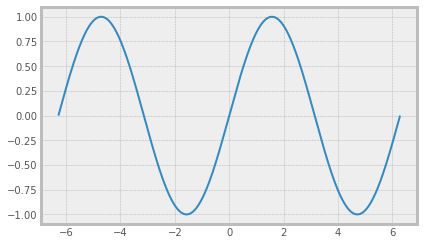To get rid of that extra line at the top, we use the show command. This is sort of like the difference between using print vs just making a variable the last line.

In :
x = 5
x

Out:
5
In :
x = np.linspace(-2*pi, 2*pi, 500)
y = np.sin(x)
plt.plot(x,y)
plt.show()## Changing the Look and Feel¶

You may switch the look and feel of plots by using the plt.style.use command. You may change the size by using the plt.figure(figsize=(4,4)) command, where you give the figure size in inches.

In :
plt.style.use('ggplot')
plt.figure(figsize=(8,6))

x = np.linspace(-2*pi, 2*pi, 500)
y = np.sin(x)
plt.plot(x,y)
plt.show()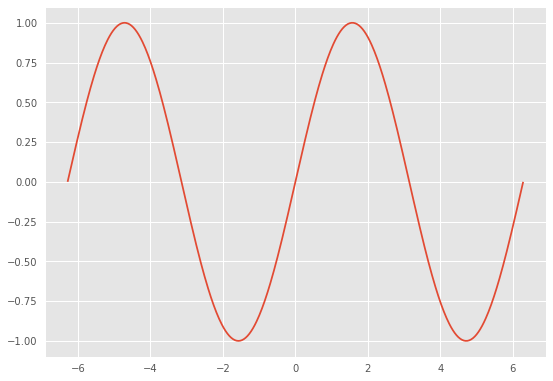In :
plt.style.use('fivethirtyeight')
plt.figure(figsize=(4,3))

x = np.linspace(-2*pi, 2*pi, 500)
y = np.sin(x)
plt.plot(x,y)
plt.show()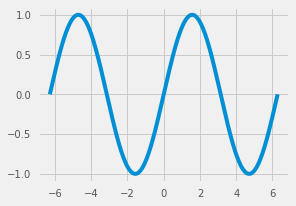In :
plt.style.available

Out:
['seaborn-colorblind',
'seaborn-muted',
'seaborn',
'seaborn-dark-palette',
'classic',
'grayscale',
'seaborn-notebook',
'seaborn-whitegrid',
'seaborn-pastel',
'seaborn-white',
'seaborn-poster',
'seaborn-dark',
'bmh',
'dark_background',
'seaborn-paper',
'ggplot',
'_classic_test',
'fast',
'Solarize_Light2',
'tableau-colorblind10',
'seaborn-deep',
'seaborn-bright',
'seaborn-talk',
'seaborn-darkgrid',
'fivethirtyeight',
'seaborn-ticks']

## Customizing plots¶

You should read the matplotlib tutorial online, but here's some basic info.

In :
plt.style.use('seaborn-whitegrid')
plt.figure(figsize=(4,3))

plt.plot(x,y)
plt.xlabel("The x-axis")
plt.ylabel("The y-axis")
plt.title("The title")
plt.show()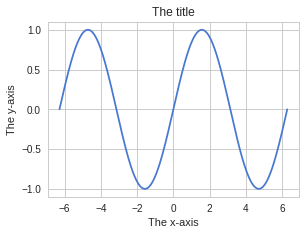In :
plt.plot(x,y, label="A sine wave")
plt.plot(x, np.cos(x), label="A cosine wave")
plt.title("A Title!")
plt.legend(loc='lower left')
plt.show()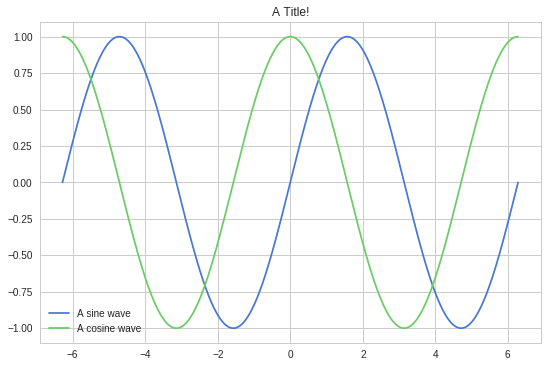In :
x = np.arange(10)
p = 0.2
geo_p = (1 - p)**(x-1) * p
plt.plot(x,geo_p,'o') #use circles
plt.show()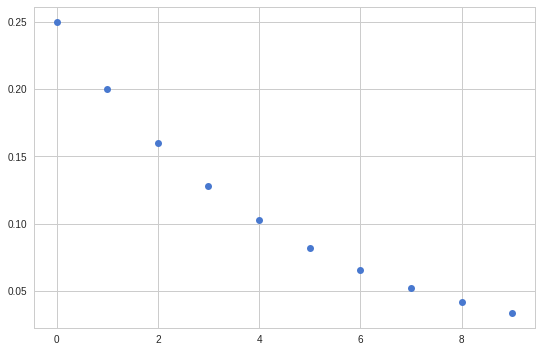In :
x = np.arange(10)
p = 0.2
geo_p = (1 - p)**(x-1) * p
plt.plot(x,geo_p,'yo-') #use yellow circles with dashes
plt.show()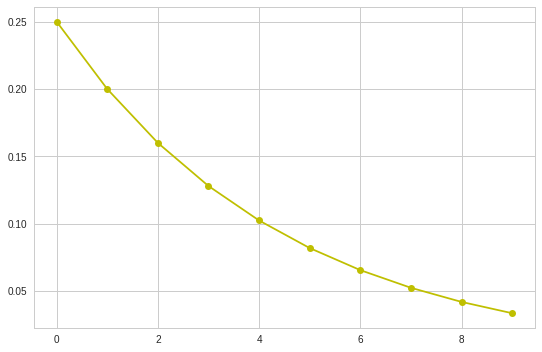## Specifying Color¶

One of the most common ways of specifying color is the default "color cycler". Matplotlib has a default set of categorical colors that represent different line types. You can specify them by using C0 or C1 or C2 etc. Let's see an example of way you might want to do this:

In :
x = np.arange(1, 10)
p = 0.2
geo_p = (1 - p)**(x-1) * p
plt.plot(x, geo_p,color='C0', label='$E_1[x]$')

#plot the mean as vertical line
plt.axvline(x=1 / p, color='C0', linestyle='--', label='$E_1[x]$')

#create a different geometric
p = 0.4
geo_p = (1 - p)**(x-1) * p
plt.plot(x, geo_p,color='C1', label='$P_2(x)$')

#plot the mean as vertical line
plt.axvline(x=1 / p, color='C1', linestyle='--', label='$E_2[x]$')

#add legend, which uses the labels = .. from above
plt.legend()

plt.show()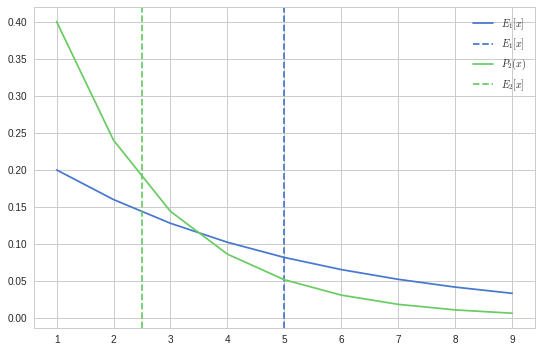Notice how I can use consistent colors for related lines in the figure. Categorical colors are for data which has no ordering. Gradient colors are for when a color has order. We will see those later.

# For Loops¶

Now that we have arrays and lists, we need need new flow statements to do something with them

In :
x = [4,3,24,7]
for element in x:
print(element)

4
3
24
7

In :
x = [4,3,24,7]
xsum = 0
for element in x:
xsum += element # <--- this means xsum = xsum + element
print(xsum)

38

In :
x = [4,3,24,7]
xsum = 0
for element in x:
xsum += element
print(xsum)

4
7
31
38


## Python Tutor¶

Python Tutor Allows you to see your code as it executes. Let's look at the last example with it.

In :
from IPython.display import HTML, display
from IPython.core.magic import register_line_cell_magic
import urllib

@register_line_cell_magic
def tutor(line, cell):
code = urllib.parse.urlencode({"code": cell})
display(HTML("""
<iframe width="800" height="500" frameborder="0"
src="http://pythontutor.com/iframe-embed.html#{}&py=2">
</iframe>
""".format(code)))

In :
%%tutor

prod = 1
for i in range(5):
prod *= i
if prod == 0:
prod = 1
print('{}! = {}'.format(i, int(prod)))


## Python Data Types¶

Let's put all we know about python data types in one place. A data type is something which can be assigned to a variable. For example, a floating point number or a string.

Here's a list of python data types we'll cover in the class:

• floating point numbers
• integers
• strings
• lists
• dictionaries
• tuples
• NumPy arrays

## Floating Points and Integers¶

In :
#Floating Point
a = 4.4
a

Out:
4.4
In :
#Converting a floating point to integer
a = int(4.4)
a

Out:
4

Converting floating points is often needed when you want to slice a list. For example

In :
a = 'A string is a list'
length = len(a)
half_length = length / 2
print(a[half_length:])

---------------------------------------------------------------------------
TypeError                                 Traceback (most recent call last)
<ipython-input-86-7c5a81e5dd65> in <module>
2 length = len(a)
3 half_length = length / 2
----> 4 print(a[half_length:])

TypeError: slice indices must be integers or None or have an __index__ method
In :
half_length = int(length / 2)
a[half_length:]

Out:
'is a list'

This is such a common occurence though that there is a shortcut to ensure that division results in an integer:

In :
3 / 2

Out:
1.5
In :
3 // 2

Out:
1
In :
a[len(a) // 2:]

Out:
'is a list'

What are the mathematical consequences of int?

In :
print(int(4.9))

4


Often that's not exactly what we want, so we can use a few functions from math

In :
from math import floor, ceil

In :
print(floor(5.9))

5

In :
print(ceil(4.0001))

5


## Dictionaries¶

Dictionaries are very similar to lists. Let's see how they work:

In :
d = dict()
d['thingie'] = 45
d['other thingie'] = 434343

print(d['thingie'])

45

In :
print(d)

{'thingie': 45, 'other thingie': 434343}


Python prints it in the format above, you can also define it using that format

In :
d = {'thing1': 3, 'b': 'a string'}
print(d['b'])

a string


We're learning dictionaries because they are often required for plotting and optimization. We'll see that later in this lecture

## Tuples¶

Tuples are just like lists, except they can't have individual elements modified.

In :
a = [0,1,2,3] # a list
a = 'fdsa'
print(a)

[0, 1, 2, 'fdsa']

In :
a = (0,1,2,3)
a = 'fdsa'

---------------------------------------------------------------------------
TypeError                                 Traceback (most recent call last)
<ipython-input-126-1a62624cd994> in <module>
1 a = (0,1,2,3)
----> 2 a = 'fdsa'

TypeError: 'tuple' object does not support item assignment

The most common place you'll see tuples is in creating numpy arrays

In :
my_tuple = (5,4)
a = np.ones(my_tuple)
print(a)

[[1. 1. 1. 1.]
[1. 1. 1. 1.]
[1. 1. 1. 1.]
[1. 1. 1. 1.]
[1. 1. 1. 1.]]


Notice that's the same as this:

In :
a = np.ones( (5,4) )
print(a)

[[1. 1. 1. 1.]
[1. 1. 1. 1.]
[1. 1. 1. 1.]
[1. 1. 1. 1.]
[1. 1. 1. 1.]]


## Function Arguments¶

Functions are a little more complicated than just the paranthesis part. There are three things you should know about functions:

1. Arguments are separated by ,
2. Arguments may be named -> foo(1,2,4, example=5)
3. You may have to pass in lists or dictionaries -> np.ones( (5,4) ) or foo(special_arg={'a':4, 'b':4})

Plotting provides some examples of this. Let's see them in action

In :
x = np.linspace(0,10, 100)
y = x**2

In :
plt.plot(x, y)
plt.text(2, 40, '$y = x^2$', fontdict={'fontsize': 24})
plt.show()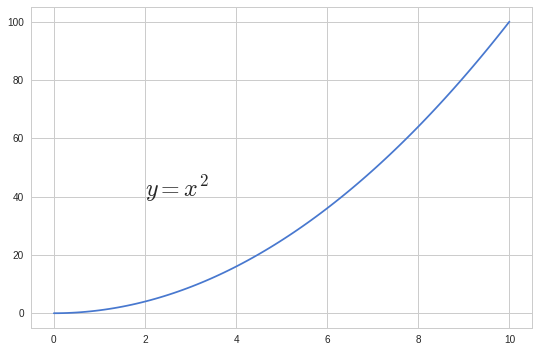Most arguments are optional. For example:

In :
from math import log
log(10)

Out:
2.302585092994046
In :
log(10, 10)

Out:
1.0

# More about Notebooks¶

## How to get help about functions¶

To get help about a particular function, type help( fxn_name ). This will post it in the output. You can instead, type fxn_name? to get a popup with help. The most useful thing though is to type SHIFT-TAB to get a tooltip about a function. To get suggestions, press TAB.

## What exactly is saved for a notebook?¶

A notebook is just a JSON file. You can open one and see this:

 {
"cell_type": "markdown",
"metadata": {},
"source": [
"To get help about a particular function, type help( fxn_name ) . This will post it in the output. You can instead, type fxn_name? to get a popup with help. The most useful thing though is to type shift-tab to get a tooltip about a function."
]
},

Jupyter will save checkpoints whenever you save. These can be accessed at file->revert-to-checkpoint. Pay close attention to the top messages to make sure you are saving your notebook. In an emergency, like your notebook failed to save, go to file->download as->jupyter notebook and it will appear in your downloads folder.

## Rescuing Python Kernel¶

It's easy to write code that will rek your kernel. You'll see this if the circle indicator in the top right corner is solid. If pressing interrupt (stop button) a few times doesn't work, save your notebook and then close the command line/terminal window and reopen one.